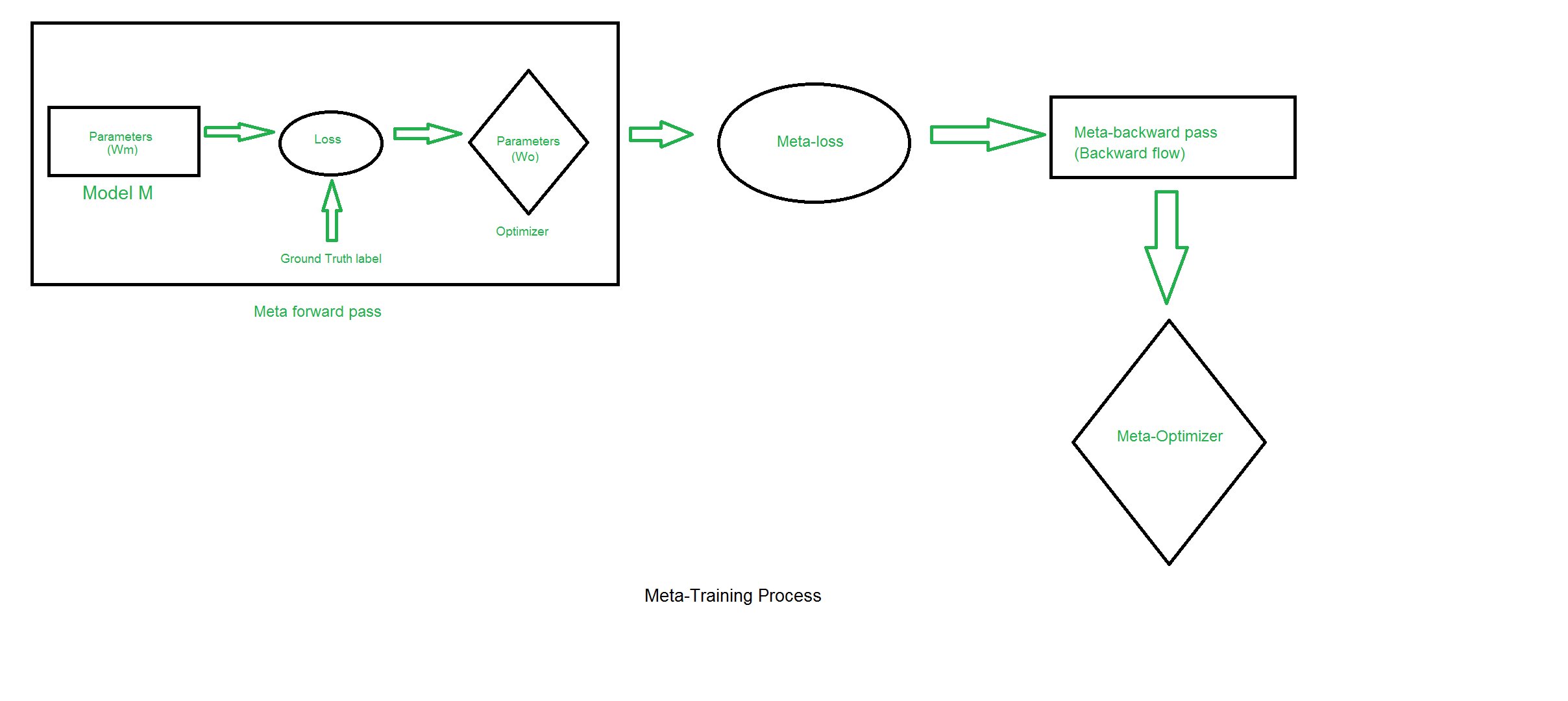Learning to learn Artificial Intelligence | An overview of Meta-Learning

• Last Updated : 19 May, 2019

What is Meta-Learning?
In traditional Machine Learning domains, we usually take a huge dataset which is specific to a particular task and wish to train a model for regression/classification purposes using this dataset. That’s radically far from how humans take advantage of their past experiences to learn very quickly a new task from only a handset of examples.

Meta-Learning is essentially learning to learn.
Formally, it can be defined as using metadata of an algorithm or a model to understand how automatic learning can become flexible in solving learning problems, hence to improve the performance of existing learning algorithms or to learn (induce) the learning algorithm itself.

Each learning algorithm is based on a set of assumptions about the data, which is called its inductive bias.
Meta-Learning takes advantage of the metadata like algorithm properties (performance measures and accuracy), or patterns previously derived from the data, to learn, select, alter or combine different learning algorithms to effectively solve a given learning problem.

The process of learning to learn or the meta-training process can be crudely summed up in the following diagram –Learning the meta-parameters-
One way to learn would be to make use of the famous Backpropagation algorithm. We can back-propagate gradient of a meta-loss along the entire training process, all the way back to the initial weights of the model.
Back-propagating the meta-loss through the model’s gradients involves computing derivatives of derivative, i.e. second derivatives which can often be computationally intensive (adding to the complexity of a meta-learning model). Popular deep learning frameworks like PyTorch and Tensorflow provide these functionalities.

To get an error value, we can simply compare the predictions of our model to the ground truth label. We also require an indicative measure of how well our meta-learner is performing i.e training the model itself.

One way to find the meta-loss can be to combine the losses of the model that we compute during the training (one possible way of combination can be just summing these losses).
Optimizers like SGD, RMSProp, Adam can be used as meta-optimizers to update the parameters (essentially the learning part of the algorithm).

Three main steps subsumed in meta-learning are –

1. Inclusion of a learning sub-model.
2. A dynamic inductive bias: Altering the inductive bias of a learning algorithm to match the given problem. This is done by altering key aspects of the learning algorithm, such as the hypothesis representation, heuristic formulae, or parameters. Many different approaches exist.
3. Extracting useful knowledge and experience from the metadata of the model: Metadata consists of knowledge about previous learning episodes and is used to efficiently develop an effective hypothesis for a new task. This is also a form of Inductive transfer.

AI can master some really complex tasks but they require massive amounts of data and are terrible at multi-tasking. So it’s important for AI agents to “learn how to learn” to gather more knowledge and become defter.

Now, let’s discuss some types of meta-learning algorithms currently found in the literature.

Types of Meta-Learning Algorithms –

1. Optimizer Meta-Learning:
This approach focuses on optimizing the entire neural network to better accomplish a task. Usually, multiple neural networks are used. One neural net is responsible for the optimization (different techniques can be used) of hyperparameters of another neural net to improve its performance.
This paper comes under the category of optimizer meta-learning, it aims to improve the performance of gradient descent.
2. Few shot learning:
Few shot learning which is a superset of many up and coming algorithms like one shot learning and zero shot learning could be the future of AI as it aims to learn by looking at only a minimal amount of data or examples. Analogously, humans also try to deduce how something works by looking at one or two instances of a problem, few shot learning aims to do the same and is a popular meta-learning algorithm.
Memory augmented neural networks and One-shot generative models come under this category. Many approaches are used for few shot learning, perhaps one of the more well-known ones is that of generating pseudo-examples to improve learning.
3. Meta-Learning applied on metrics:
This approach basically aims to find a metric space in which learning is more effective and efficient.
This paper is a good fit for this category. Also, it can be observed that this category is a subset of the few-shot learning approach.
4. Model-Agnostic Meta-Learning:
Introduced recently, in 2017 by Finn et al., Model-agnostic Meta-learning (MAML) has showcased brilliant performance on many tasks. The parameters of a model are trained such that even a few iterations of applying gradient descent with relatively few data samples from a new task (new domain) can lead to good generalization on that task.
It has been used in various other sub-domains like Meta-Reinforcement Learning (Policy gradient based RL) and has also set the benchmarks in computer vision tasks, especially few-shot learning in image classification.

1. Meta-Learning offers more speed: Meta-learning approaches can produce learning architectures that perform better and faster than hand-crafted models.
2. Scaling: Meta-learning can automate the process of choosing and fine-tuning algorithms, thereby increasing the potential to scale AI applications.
3. Less data required: These approaches assist in the development of more general systems, which can transfer knowledge from one context to another. This reduces the amount of data you need in solving problems in the new context.

Relevant applications:

Meta-learning algorithms are already in use in various applications, some of which are:

1. Fraudulent Transaction detection